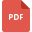# decimals and multiplication tutorialsIntroductions to Decimal Numbers
Decimals and Multiplication, Grade 5, United States, MathematicsMultiplication of Decimals by Decimals to Two Decimal Places Worksheet
Decimals and Multiplication, Grade 8, United States, MathematicsMultiplying Decimals by Whole Numbers Worksheet
Decimals and Multiplication, Grade 8, United States, MathematicsMultiplying and Dividing Decimals
Mathematics, Grade 7, United States, Decimals and Division, Decimals and Multiplication, Decimal and FractionsMultiplying and Dividing decimals by 10, 100, and 1000
Decimals and Multiplication, Grade 5, United States, Decimals and DivisionMultiplying decimals by 10, 100 and 1000
Decimals and Multiplication, Grade 6, United States